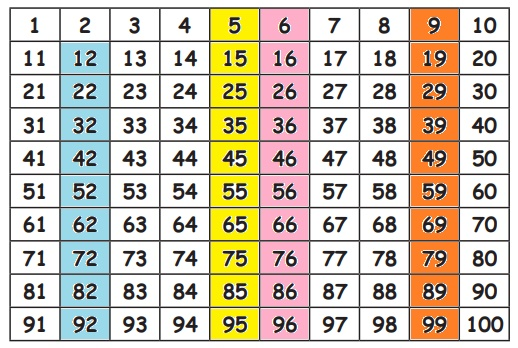Home | | Maths 3rd Std | Following and Devising Algorithms: quick way of finding

# Following and Devising Algorithms: quick way of finding

The quick way of finding 10 more than and less than a given number.

The quick way of finding 10 more than and less than a given number.

Colour the table in the next page by skip counting in tens as per the instructions given below.

1. Colour the numbers starting from 12 in blue.

2. Colour the numbers starting from 6 in pink.

3. Colour the numbers starting from 5 in yellow.

4. Colour the numbers starting from 9 in orange.

After colouring observe the table and fill in the blanks.1. 10 more than 45 is 55.

2. 10 less than 45 is 35.

3. 10 more than 22 is 32.

4. 10 less than 22 is 12.

Activity

Complete the blanksTags : Information Processing | Term 2 Chapter 5 | 3rd Maths , 3rd Maths : Term 2 Unit 5 : Information Processing
Study Material, Lecturing Notes, Assignment, Reference, Wiki description explanation, brief detail
3rd Maths : Term 2 Unit 5 : Information Processing : Following and Devising Algorithms: quick way of finding | Information Processing | Term 2 Chapter 5 | 3rd Maths